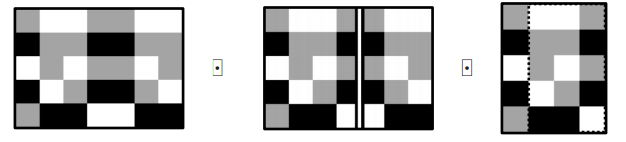시간 제한 메모리 제한 제출 정답 맞은 사람 정답 비율
1 초 256 MB 2 2 2 100.000%

## 문제

You are given a piece of paper in the shape of a matrix of size $N \times M$. Every cell is colored in one of 26 possible colors which are represented by lowercase letters of the English alphabet. The piece of paper can be folded in the following way. First, you choose a vertical line between two of its columns or a horizontal line between two of its rows. This line splits the paper into two sides. You then use the line as the folding axis and fold the smaller side of the paper onto the larger one going over the axis.  However, you can only do this if all the cells of the smaller side of the paper have matching colors with their corresponding cells on the other side (in other words, each cell should have the same color as its reflection over the chosen axis). If both sides of the paper are of equal size, you may fold from either side.

You notice that after any number of folding operations, you end up with a contiguous submatrix of the original piece of paper. How many different submatrices of the initial piece of paper can you obtain by doing arbitrarily many folding operations (possibly none)? Two submatrices are considered different if they occupy different coordinates in the initial matrix, even if they have identical color content.## 입력

The first line of input contains two integers $N$ and $M$ ($N, M \ge 1$, $1 \le N \cdot M \le 1\,000\,000$).

Each of the next $N$ lines contains a string of length $M$ consisting of lowercase English letters. Together, these lines describe the given piece of paper.

## 출력

Print a single line with a single integer: the number of different submatrices that you can obtain by folding the paper.

## 예제 입력 1

5 7
baabbaa
cbbccbb
ababbab
cabccba
bccaacc


## 예제 출력 1

2